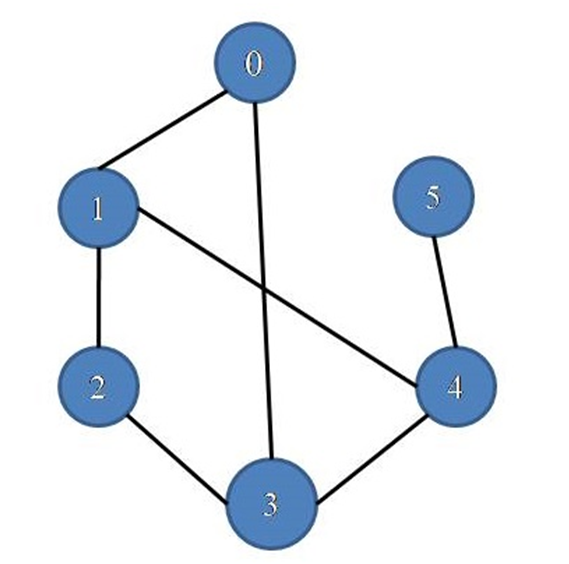# Cycle Detection in an Undirected Graph

In this article, we are going to detect cycle in an undirected graph with C++ implementation.
Submitted by Souvik Saha, on March 19, 2019

What you will learn?

How to detect a cycle in an undirected graph?

In the graph below,It has cycles 0-1-4-3-0 or 0-1-2-3-0

Algorithm:

Here we use a recursive method to detect a cycle in a graph.

1. We check the presence of a cycle starting by each and every node at a time.
2. For each node
1. Whenever we visited one vertex we mark it.
2. Except for the starting node, we store its parent node go for all its adjacent nodes.
3. If there is any marked vertex present except parent that means there is a cycle present in the graph.

Function:

```Check(parent , current node){
visit[curr_node]=true;
for( all the adjacent vertices ){
if(not visited yet) then
else if( the vertex is not the parent) then
There is a cycle in the graph
}
}
```

C++ implementation:

```#include <bits/stdc++.h>
using namespace std;

void cycle_check(list<int>*,int);

//	Make a pair between vertex x and vertex y
ls[x].push_back(y);
ls[y].push_back(x);
return;
}

void check_cycle_util(list<int> *ls,bool *visit,int curr_node,int parent,int &temp){
visit[curr_node]=true;
list<int>::iterator it;
for(it=ls[curr_node].begin();it!=ls[curr_node].end();it++){
if(!visit[*it]){
check_cycle_util(ls,visit,*it,curr_node,temp);
}
else if(*it != parent && temp==0){
temp=1;
cout<<"There is a cycle in the graph\n";
break;
}
}
}

//checking the cycles in a graph
void cycle_check(list<int>*ls,int num){
bool *visit=new bool[num];
int temp=0;
for(int i=0;i<num;i++)
visit[i]=false;
for(int i=0;i<num;i++){
if(!visit[i] && temp==0){
check_cycle_util(ls,visit,i,-2,temp);
}
}
}

int main(){
int num;

cout<<"Enter the no. of vertices :";
cin>>num;

list<int> *ls=new list<int>[num];
cycle_check(ls,6);

return 0;
}
```

Output

```Enter the no. of vertices : 6
There is a cycle in the graph
```

Top MCQs

Languages: » C » C++ » C++ STL » Java » Data Structure » C#.Net » Android » Kotlin » SQL
Web Technologies: » PHP » Python » JavaScript » CSS » Ajax » Node.js » Web programming/HTML
Solved programs: » C » C++ » DS » Java » C#
Aptitude que. & ans.: » C » C++ » Java » DBMS
Interview que. & ans.: » C » Embedded C » Java » SEO » HR
CS Subjects: » CS Basics » O.S. » Networks » DBMS » Embedded Systems » Cloud Computing
» Machine learning » CS Organizations » Linux » DOS
More: » Articles » Puzzles » News/Updates